Скачать презентацию This Week v Liquids and Gases v Pressure

07b6f8f1f02e264fb2fd91134427fa83.ppt

• Количество слайдов: 47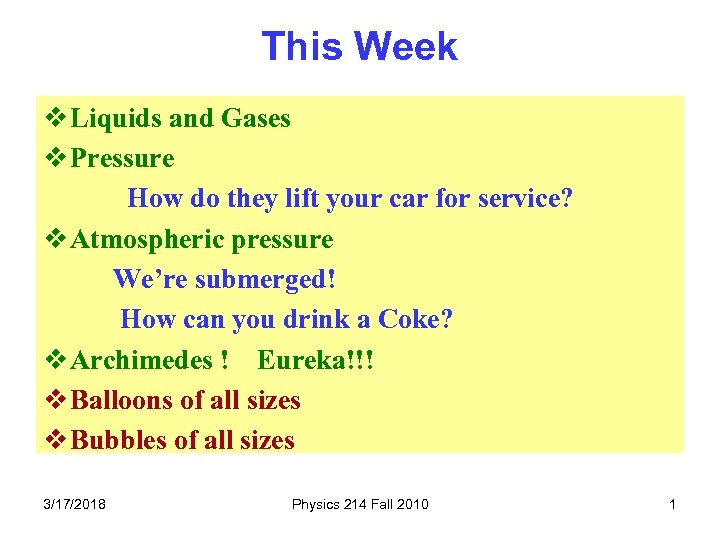This Week v Liquids and Gases v Pressure How do they lift your car for service? v Atmospheric pressure We’re submerged! How can you drink a Coke? v Archimedes ! Eureka!!! v Balloons of all sizes v Bubbles of all sizes 3/17/2018 Physics 214 Fall 2010 1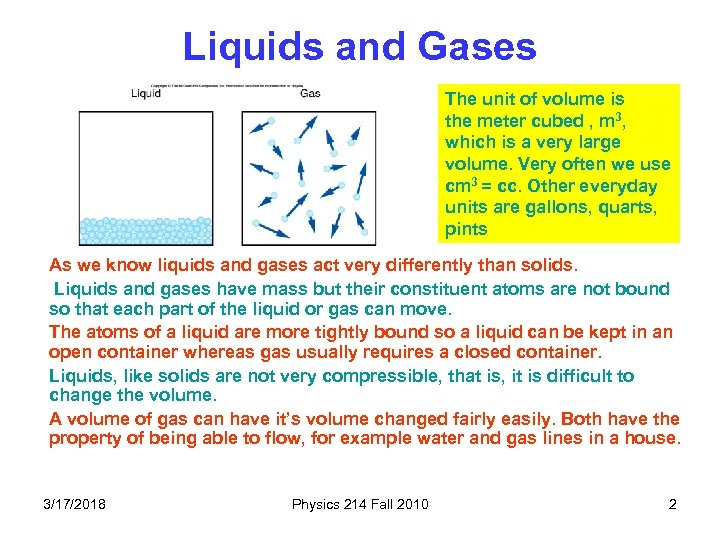Liquids and Gases The unit of volume is the meter cubed , m 3, which is a very large volume. Very often we use cm 3 = cc. Other everyday units are gallons, quarts, pints As we know liquids and gases act very differently than solids. Liquids and gases have mass but their constituent atoms are not bound so that each part of the liquid or gas can move. The atoms of a liquid are more tightly bound so a liquid can be kept in an open container whereas gas usually requires a closed container. Liquids, like solids are not very compressible, that is, it is difficult to change the volume. A volume of gas can have it’s volume changed fairly easily. Both have the property of being able to flow, for example water and gas lines in a house. 3/17/2018 Physics 214 Fall 2010 2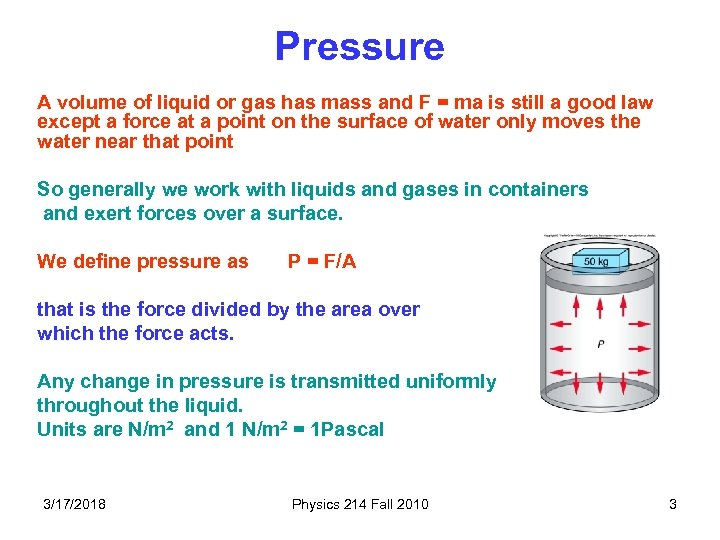Pressure A volume of liquid or gas has mass and F = ma is still a good law except a force at a point on the surface of water only moves the water near that point So generally we work with liquids and gases in containers and exert forces over a surface. We define pressure as P = F/A that is the force divided by the area over which the force acts. Any change in pressure is transmitted uniformly throughout the liquid. Units are N/m 2 and 1 N/m 2 = 1 Pascal 3/17/2018 Physics 214 Fall 2010 3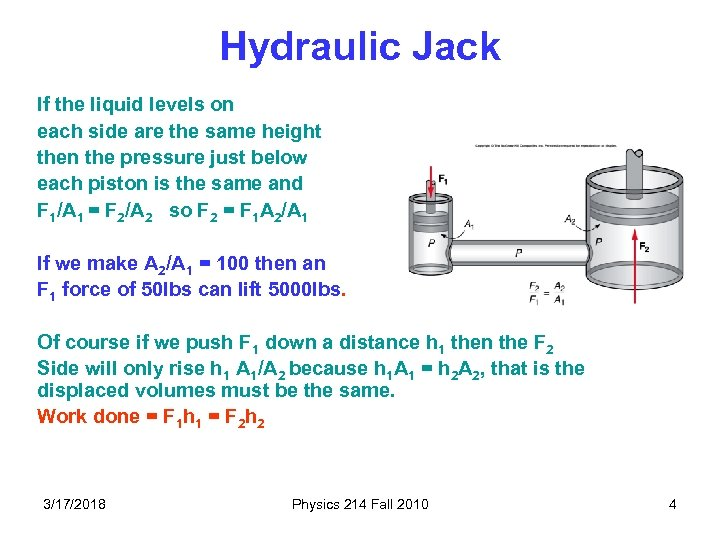Hydraulic Jack If the liquid levels on each side are the same height then the pressure just below each piston is the same and F 1/A 1 = F 2/A 2 so F 2 = F 1 A 2/A 1 If we make A 2/A 1 = 100 then an F 1 force of 50 lbs can lift 5000 lbs. Of course if we push F 1 down a distance h 1 then the F 2 Side will only rise h 1 A 1/A 2 because h 1 A 1 = h 2 A 2, that is the displaced volumes must be the same. Work done = F 1 h 1 = F 2 h 2 3/17/2018 Physics 214 Fall 2010 4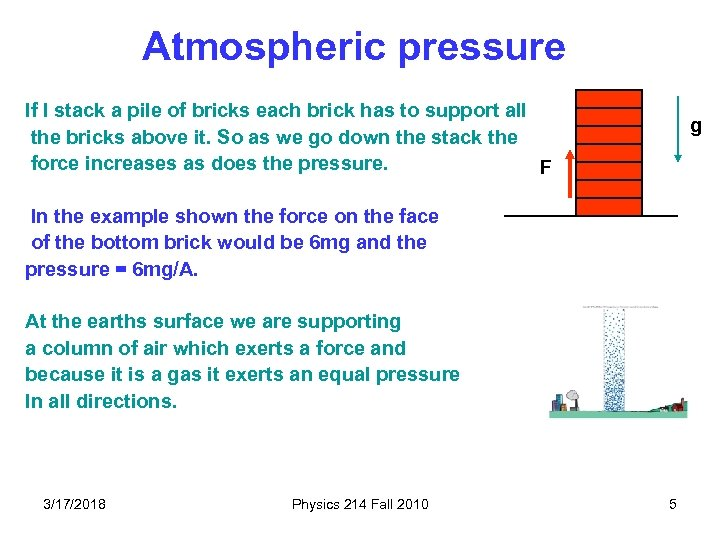Atmospheric pressure If I stack a pile of bricks each brick has to support all the bricks above it. So as we go down the stack the force increases as does the pressure. F g In the example shown the force on the face of the bottom brick would be 6 mg and the pressure = 6 mg/A. At the earths surface we are supporting a column of air which exerts a force and because it is a gas it exerts an equal pressure In all directions. 3/17/2018 Physics 214 Fall 2010 5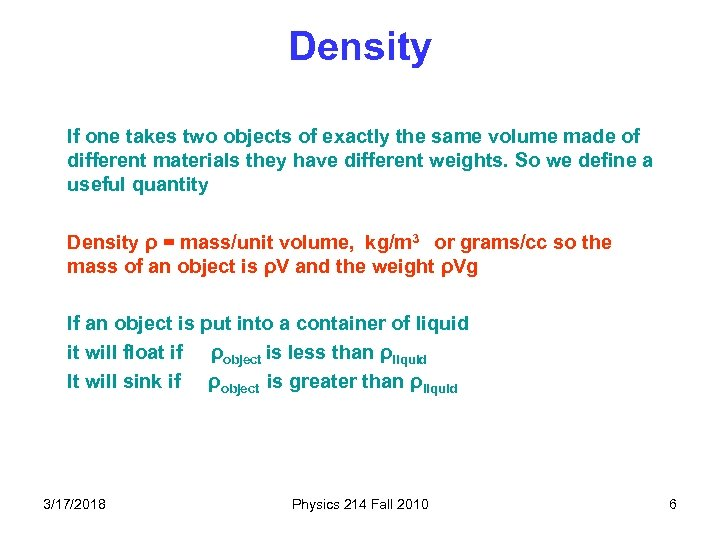Density If one takes two objects of exactly the same volume made of different materials they have different weights. So we define a useful quantity Density ρ = mass/unit volume, kg/m 3 or grams/cc so the mass of an object is ρV and the weight ρVg If an object is put into a container of liquid it will float if ρobject is less than ρliquid It will sink if ρobject is greater than ρliquid 3/17/2018 Physics 214 Fall 2010 6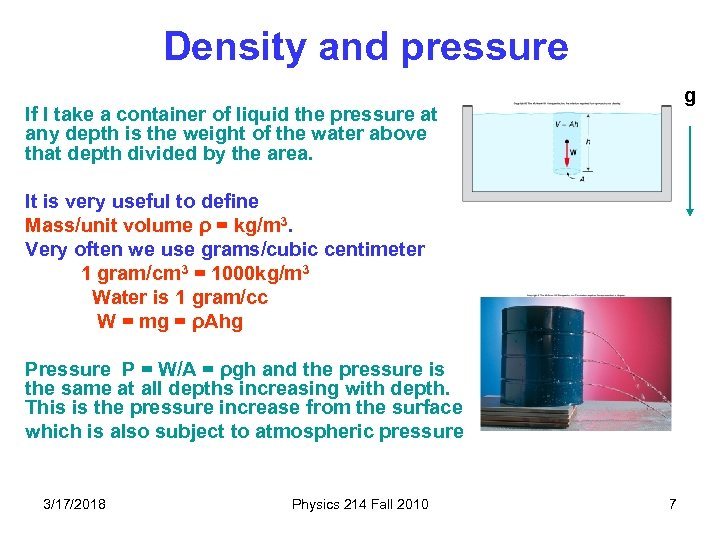Density and pressure g If I take a container of liquid the pressure at any depth is the weight of the water above that depth divided by the area. It is very useful to define Mass/unit volume ρ = kg/m 3. Very often we use grams/cubic centimeter 1 gram/cm 3 = 1000 kg/m 3 Water is 1 gram/cc W = mg = ρAhg Pressure P = W/A = ρgh and the pressure is the same at all depths increasing with depth. This is the pressure increase from the surface which is also subject to atmospheric pressure 3/17/2018 Physics 214 Fall 2010 7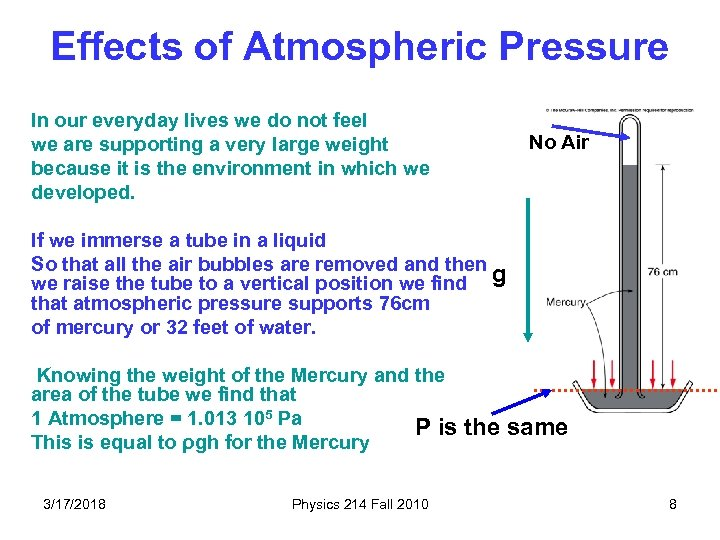Effects of Atmospheric Pressure In our everyday lives we do not feel we are supporting a very large weight because it is the environment in which we developed. No Air If we immerse a tube in a liquid So that all the air bubbles are removed and then we raise the tube to a vertical position we find g that atmospheric pressure supports 76 cm of mercury or 32 feet of water. Knowing the weight of the Mercury and the area of the tube we find that 1 Atmosphere = 1. 013 105 Pa P is This is equal to ρgh for the Mercury 3/17/2018 Physics 214 Fall 2010 the same 8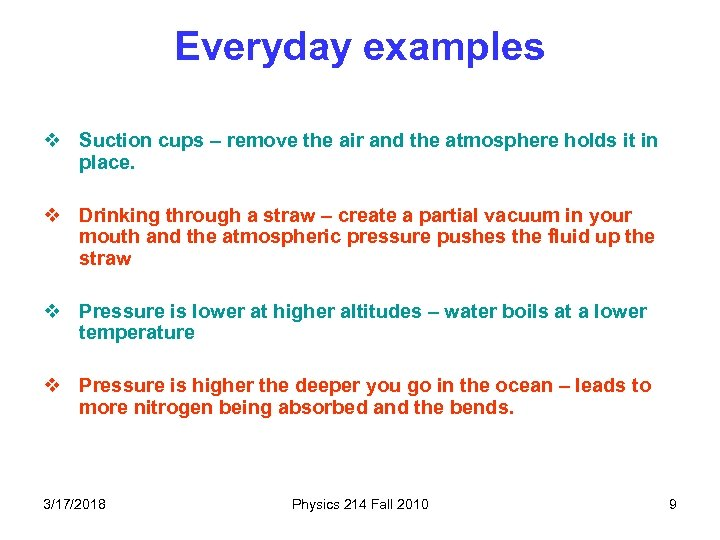Everyday examples v Suction cups – remove the air and the atmosphere holds it in place. v Drinking through a straw – create a partial vacuum in your mouth and the atmospheric pressure pushes the fluid up the straw v Pressure is lower at higher altitudes – water boils at a lower temperature v Pressure is higher the deeper you go in the ocean – leads to more nitrogen being absorbed and the bends. 3/17/2018 Physics 214 Fall 2010 9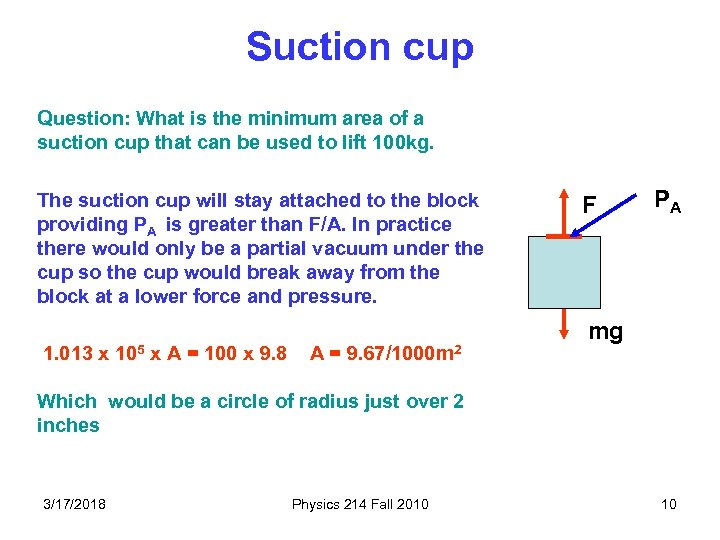Suction cup Question: What is the minimum area of a suction cup that can be used to lift 100 kg. The suction cup will stay attached to the block providing PA is greater than F/A. In practice there would only be a partial vacuum under the cup so the cup would break away from the block at a lower force and pressure. 1. 013 x 105 x A = 100 x 9. 8 A = 9. 67/1000 m 2 F PA mg Which would be a circle of radius just over 2 inches 3/17/2018 Physics 214 Fall 2010 10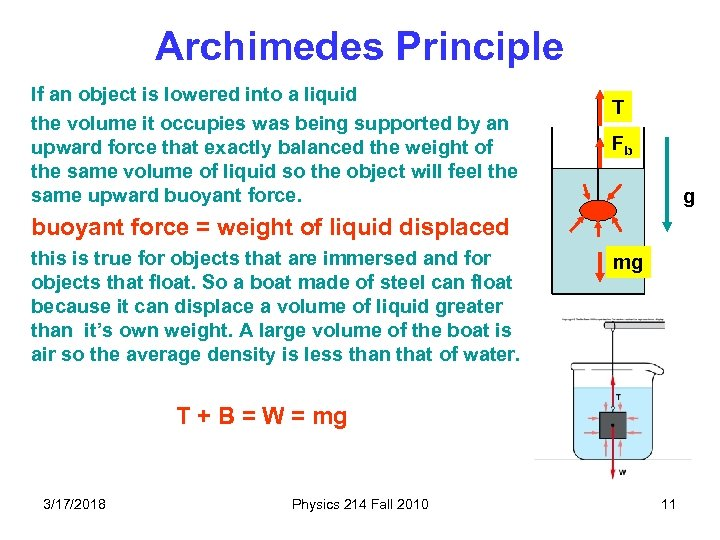Archimedes Principle If an object is lowered into a liquid the volume it occupies was being supported by an upward force that exactly balanced the weight of the same volume of liquid so the object will feel the same upward buoyant force. T Fb g buoyant force = weight of liquid displaced this is true for objects that are immersed and for objects that float. So a boat made of steel can float because it can displace a volume of liquid greater than it’s own weight. A large volume of the boat is air so the average density is less than that of water. mg T + B = W = mg 3/17/2018 Physics 214 Fall 2010 11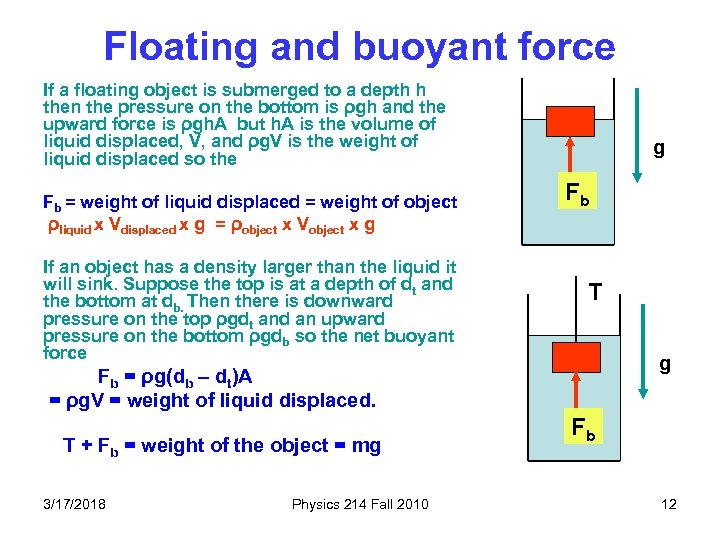Floating and buoyant force If a floating object is submerged to a depth h then the pressure on the bottom is ρgh and the upward force is ρgh. A but h. A is the volume of liquid displaced, V, and ρg. V is the weight of liquid displaced so the Fb = weight of liquid displaced = weight of object ρliquid x Vdisplaced x g = ρobject x Vobject x g If an object has a density larger than the liquid it will sink. Suppose the top is at a depth of dt and the bottom at db. Then there is downward pressure on the top ρgdt and an upward pressure on the bottom ρgdb so the net buoyant force g Fb T g Fb = ρg(db – dt)A = ρg. V = weight of liquid displaced. T + Fb = weight of the object = mg 3/17/2018 Physics 214 Fall 2010 Fb 12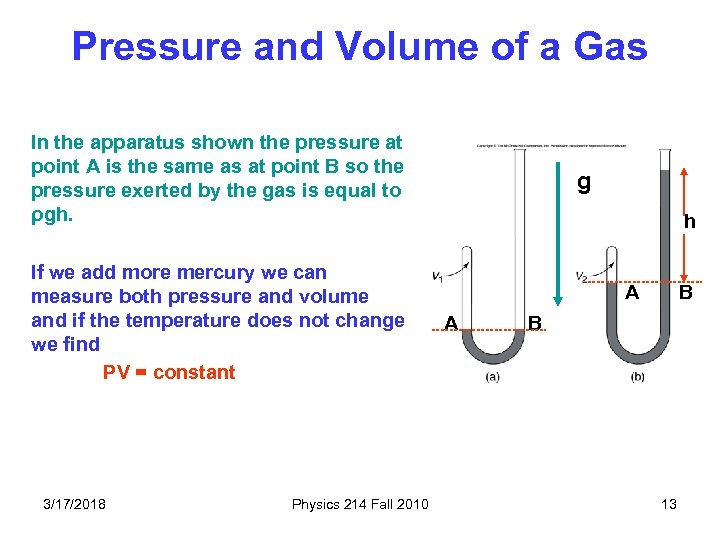Pressure and Volume of a Gas In the apparatus shown the pressure at point A is the same as at point B so the pressure exerted by the gas is equal to ρgh. If we add more mercury we can measure both pressure and volume and if the temperature does not change we find PV = constant 3/17/2018 Physics 214 Fall 2010 g h A A B B 13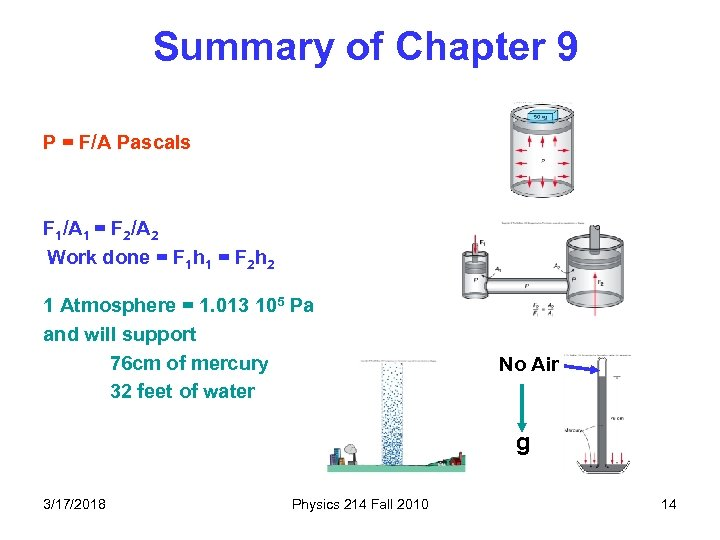Summary of Chapter 9 P = F/A Pascals F 1/A 1 = F 2/A 2 Work done = F 1 h 1 = F 2 h 2 1 Atmosphere = 1. 013 105 Pa and will support 76 cm of mercury 32 feet of water No Air g 3/17/2018 Physics 214 Fall 2010 14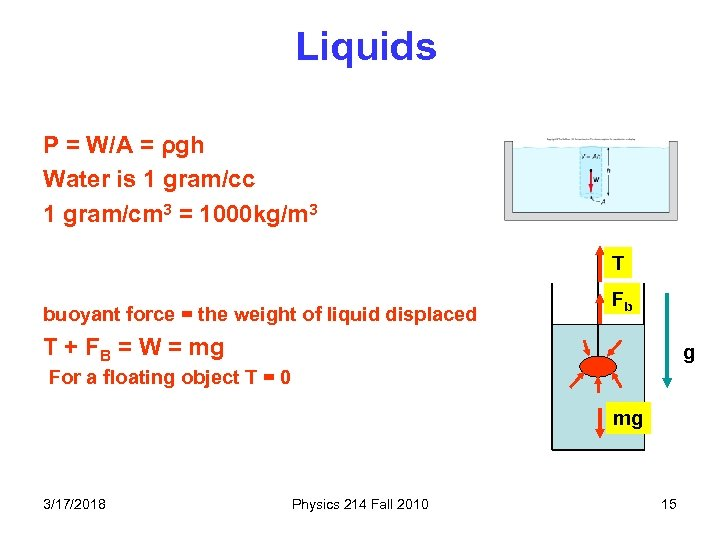Liquids P = W/A = ρgh Water is 1 gram/cc 1 gram/cm 3 = 1000 kg/m 3 T buoyant force = the weight of liquid displaced Fb T + FB = W = mg g For a floating object T = 0 mg 3/17/2018 Physics 214 Fall 2010 15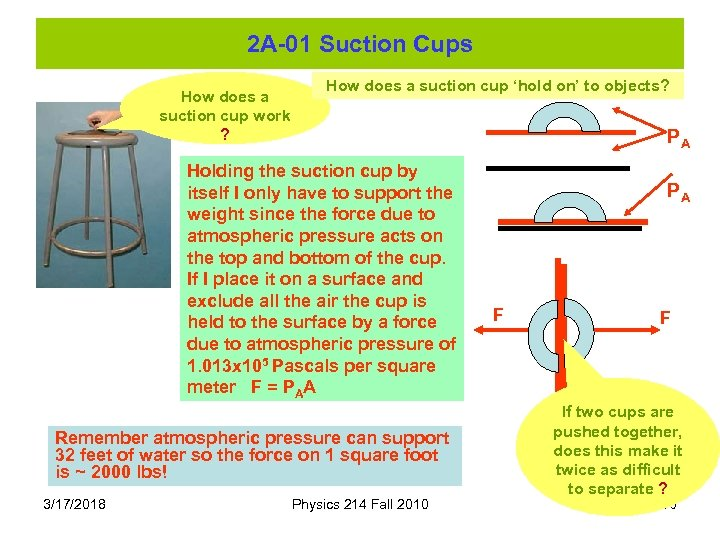2 A-01 Suction Cups How does a suction cup work ? How does a suction cup ‘hold on’ to objects? PA Holding the suction cup by itself I only have to support the weight since the force due to atmospheric pressure acts on the top and bottom of the cup. If I place it on a surface and exclude all the air the cup is held to the surface by a force due to atmospheric pressure of 1. 013 x 105 Pascals per square meter F = PAA Remember atmospheric pressure can support 32 feet of water so the force on 1 square foot is ~ 2000 lbs! 3/17/2018 Physics 214 Fall 2010 PA F F If two cups are pushed together, does this make it twice as difficult to separate ? 16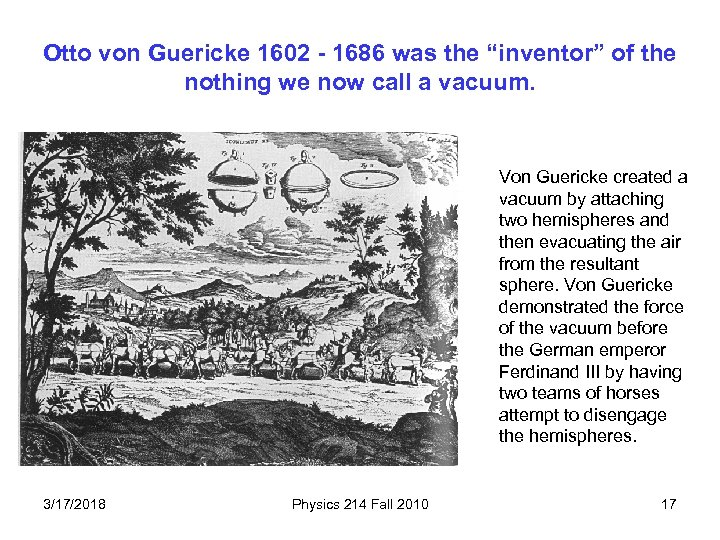Otto von Guericke 1602 - 1686 was the “inventor” of the nothing we now call a vacuum. Von Guericke created a vacuum by attaching two hemispheres and then evacuating the air from the resultant sphere. Von Guericke demonstrated the force of the vacuum before the German emperor Ferdinand III by having two teams of horses attempt to disengage the hemispheres. 3/17/2018 Physics 214 Fall 2010 17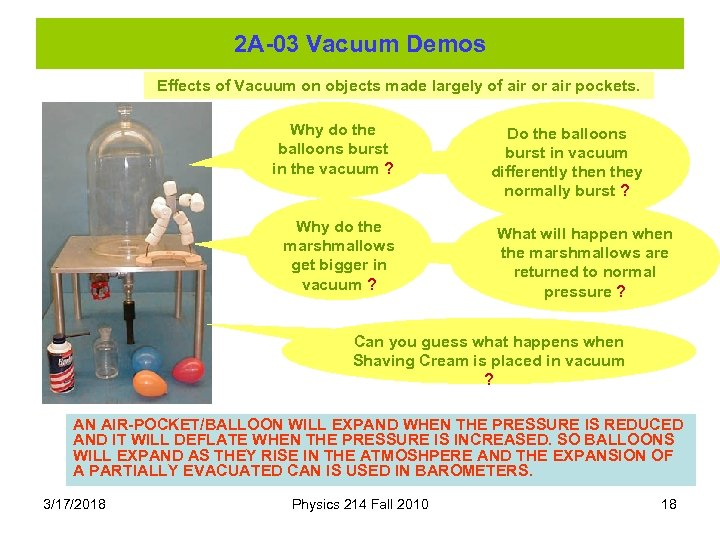2 A-03 Vacuum Demos Effects of Vacuum on objects made largely of air or air pockets. Why do the balloons burst in the vacuum ? Why do the marshmallows get bigger in vacuum ? Do the balloons burst in vacuum differently then they normally burst ? What will happen when the marshmallows are returned to normal pressure ? Can you guess what happens when Shaving Cream is placed in vacuum ? AN AIR-POCKET/BALLOON WILL EXPAND WHEN THE PRESSURE IS REDUCED AND IT WILL DEFLATE WHEN THE PRESSURE IS INCREASED. SO BALLOONS WILL EXPAND AS THEY RISE IN THE ATMOSHPERE AND THE EXPANSION OF A PARTIALLY EVACUATED CAN IS USED IN BAROMETERS. 3/17/2018 Physics 214 Fall 2010 18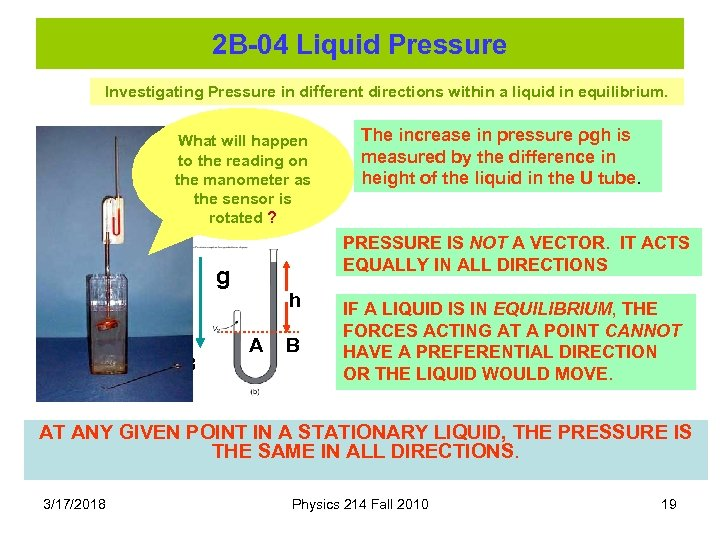2 B-04 Liquid Pressure Investigating Pressure in different directions within a liquid in equilibrium. What will happen to the reading on the manometer as the sensor is rotated ? PRESSURE IS NOT A VECTOR. IT ACTS EQUALLY IN ALL DIRECTIONS g A B The increase in pressure ρgh is measured by the difference in height of the liquid in the U tube. h A B IF A LIQUID IS IN EQUILIBRIUM, THE FORCES ACTING AT A POINT CANNOT HAVE A PREFERENTIAL DIRECTION OR THE LIQUID WOULD MOVE. AT ANY GIVEN POINT IN A STATIONARY LIQUID, THE PRESSURE IS THE SAME IN ALL DIRECTIONS. 3/17/2018 Physics 214 Fall 2010 19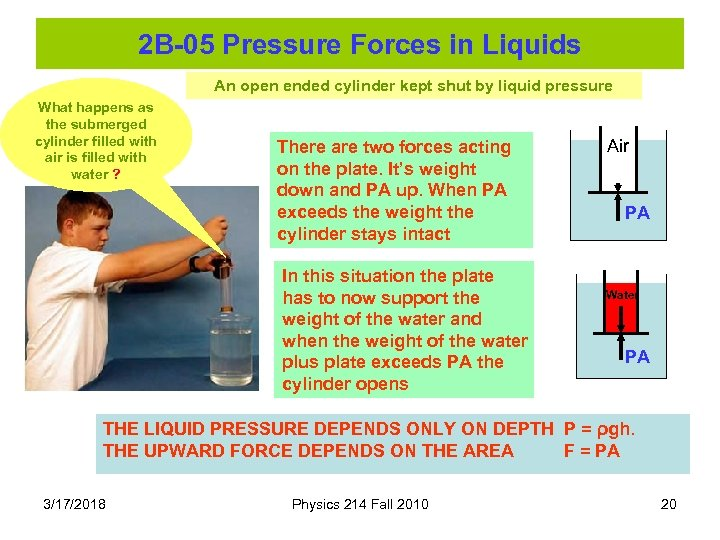2 B-05 Pressure Forces in Liquids An open ended cylinder kept shut by liquid pressure What happens as the submerged cylinder filled with air is filled with water ? There are two forces acting on the plate. It’s weight down and PA up. When PA exceeds the weight the cylinder stays intact In this situation the plate has to now support the weight of the water and when the weight of the water plus plate exceeds PA the cylinder opens Air PA Water PA THE LIQUID PRESSURE DEPENDS ONLY ON DEPTH P = ρgh. THE UPWARD FORCE DEPENDS ON THE AREA F = PA 3/17/2018 Physics 214 Fall 2010 20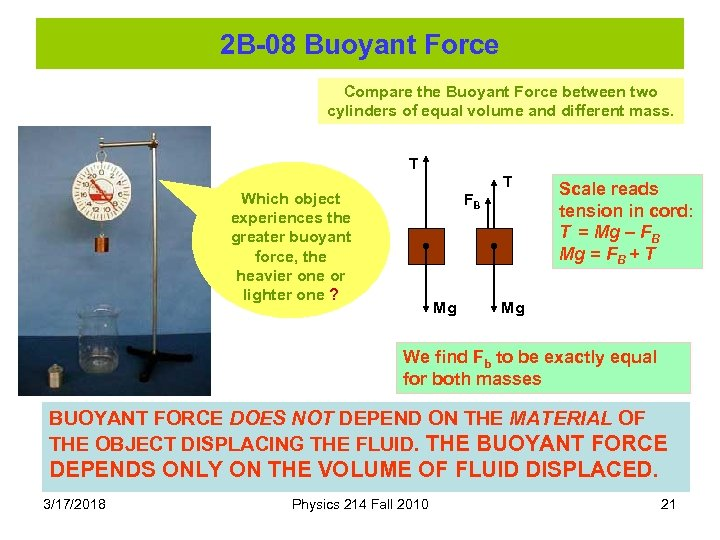2 B-08 Buoyant Force Compare the Buoyant Force between two cylinders of equal volume and different mass. T T Which object experiences the greater buoyant force, the heavier one or lighter one ? FB Mg Scale reads tension in cord: T = Mg – FB Mg = FB + T Mg We find Fb to be exactly equal for both masses BUOYANT FORCE DOES NOT DEPEND ON THE MATERIAL OF THE OBJECT DISPLACING THE FLUID. THE BUOYANT FORCE DEPENDS ONLY ON THE VOLUME OF FLUID DISPLACED. 3/17/2018 Physics 214 Fall 2010 21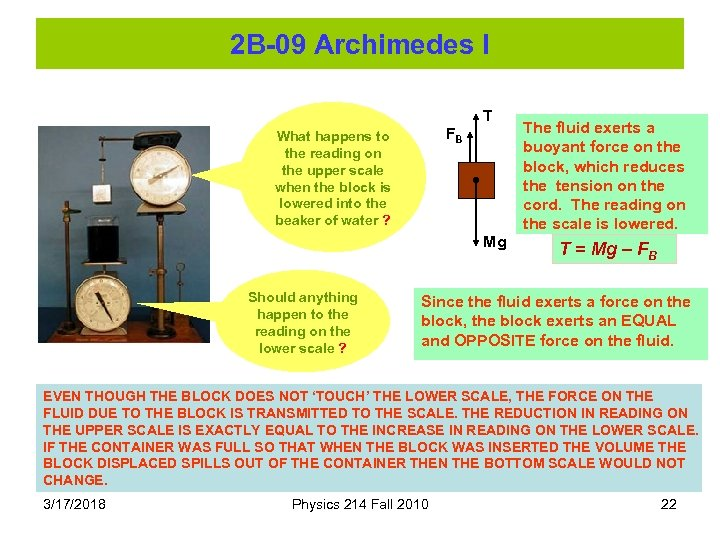2 B-09 Archimedes I T FB What happens to the reading on the upper scale when the block is lowered into the beaker of water ? Mg Should anything happen to the reading on the lower scale ? The fluid exerts a buoyant force on the block, which reduces the tension on the cord. The reading on the scale is lowered. T = Mg – FB Since the fluid exerts a force on the block, the block exerts an EQUAL and OPPOSITE force on the fluid. EVEN THOUGH THE BLOCK DOES NOT ‘TOUCH’ THE LOWER SCALE, THE FORCE ON THE FLUID DUE TO THE BLOCK IS TRANSMITTED TO THE SCALE. THE REDUCTION IN READING ON THE UPPER SCALE IS EXACTLY EQUAL TO THE INCREASE IN READING ON THE LOWER SCALE. IF THE CONTAINER WAS FULL SO THAT WHEN THE BLOCK WAS INSERTED THE VOLUME THE BLOCK DISPLACED SPILLS OUT OF THE CONTAINER THEN THE BOTTOM SCALE WOULD NOT CHANGE. 3/17/2018 Physics 214 Fall 2010 22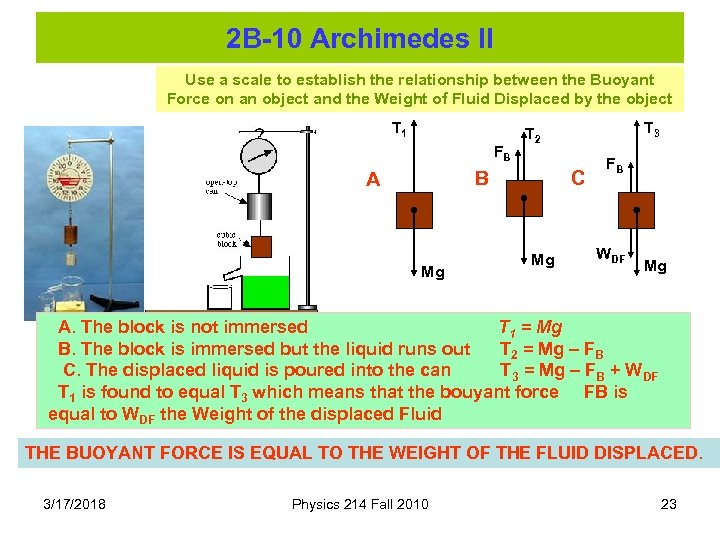2 B-10 Archimedes II Use a scale to establish the relationship between the Buoyant Force on an object and the Weight of Fluid Displaced by the object T 1 FB B A Mg T 3 T 2 C Mg FB WDF Mg A. The block is not immersed T 1 = Mg B. The block is immersed but the liquid runs out T 2 = Mg – FB C. The displaced liquid is poured into the can T 3 = Mg – FB + WDF T 1 is found to equal T 3 which means that the bouyant force FB is equal to WDF the Weight of the displaced Fluid THE BUOYANT FORCE IS EQUAL TO THE WEIGHT OF THE FLUID DISPLACED. 3/17/2018 Physics 214 Fall 2010 23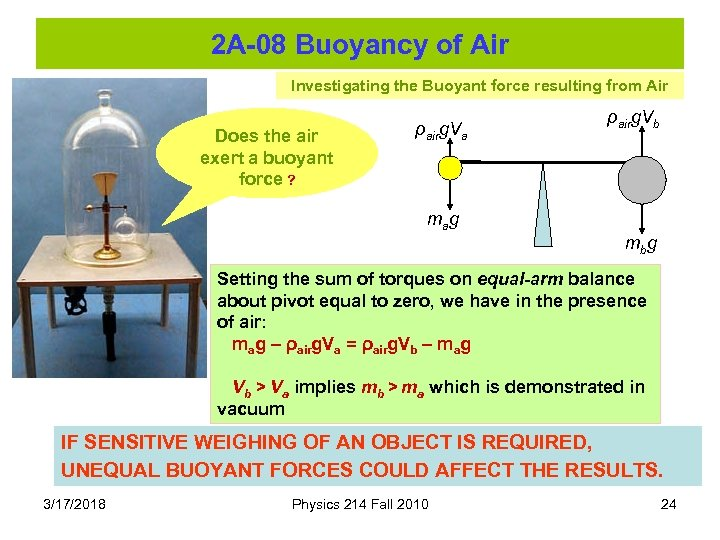2 A-08 Buoyancy of Air Investigating the Buoyant force resulting from Air Does the air exert a buoyant force ? ρairg. Va ρairg. Vb ma g mb g Setting the sum of torques on equal-arm balance about pivot equal to zero, we have in the presence of air: mag – ρairg. Va = ρairg. Vb – mag Vb > Va implies mb > ma which is demonstrated in vacuum IF SENSITIVE WEIGHING OF AN OBJECT IS REQUIRED, UNEQUAL BUOYANT FORCES COULD AFFECT THE RESULTS. 3/17/2018 Physics 214 Fall 2010 24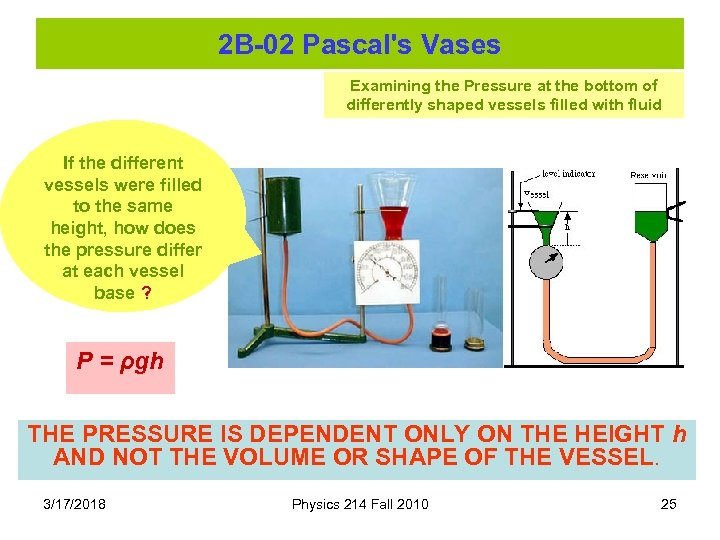2 B-02 Pascal's Vases Examining the Pressure at the bottom of differently shaped vessels filled with fluid If the different vessels were filled to the same height, how does the pressure differ at each vessel base ? P = ρgh THE PRESSURE IS DEPENDENT ONLY ON THE HEIGHT h AND NOT THE VOLUME OR SHAPE OF THE VESSEL. 3/17/2018 Physics 214 Fall 2010 25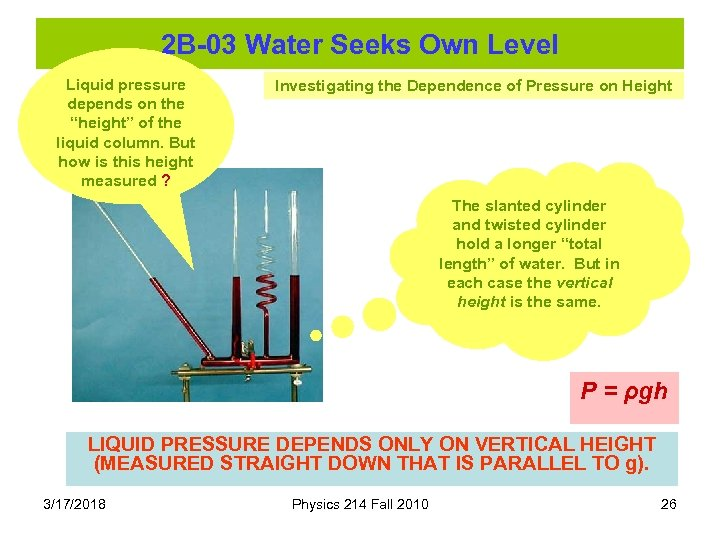2 B-03 Water Seeks Own Level Liquid pressure depends on the “height” of the liquid column. But how is this height measured ? Investigating the Dependence of Pressure on Height The slanted cylinder and twisted cylinder hold a longer “total length” of water. But in each case the vertical height is the same. P = ρgh LIQUID PRESSURE DEPENDS ONLY ON VERTICAL HEIGHT (MEASURED STRAIGHT DOWN THAT IS PARALLEL TO g). 3/17/2018 Physics 214 Fall 2010 26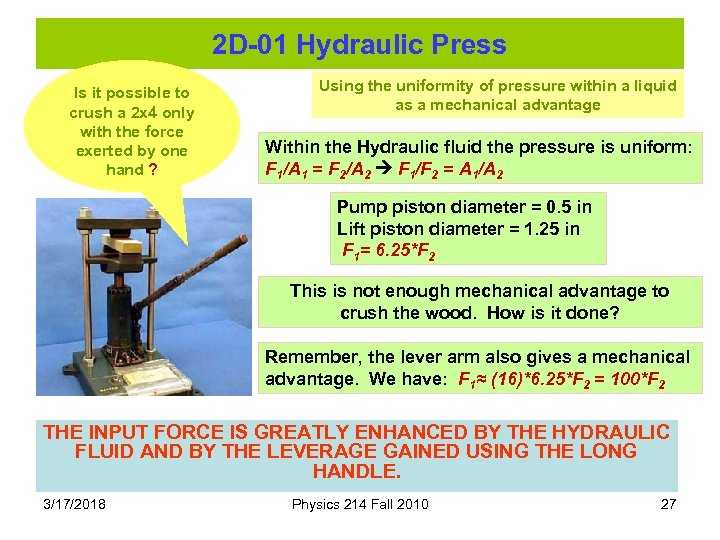2 D-01 Hydraulic Press Is it possible to crush a 2 x 4 only with the force exerted by one hand ? Using the uniformity of pressure within a liquid as a mechanical advantage Within the Hydraulic fluid the pressure is uniform: F 1/A 1 = F 2/A 2 F 1/F 2 = A 1/A 2 Pump piston diameter = 0. 5 in Lift piston diameter = 1. 25 in F 1= 6. 25*F 2 This is not enough mechanical advantage to crush the wood. How is it done? Remember, the lever arm also gives a mechanical advantage. We have: F 1≈ (16)*6. 25*F 2 = 100*F 2 THE INPUT FORCE IS GREATLY ENHANCED BY THE HYDRAULIC FLUID AND BY THE LEVERAGE GAINED USING THE LONG HANDLE. 3/17/2018 Physics 214 Fall 2010 27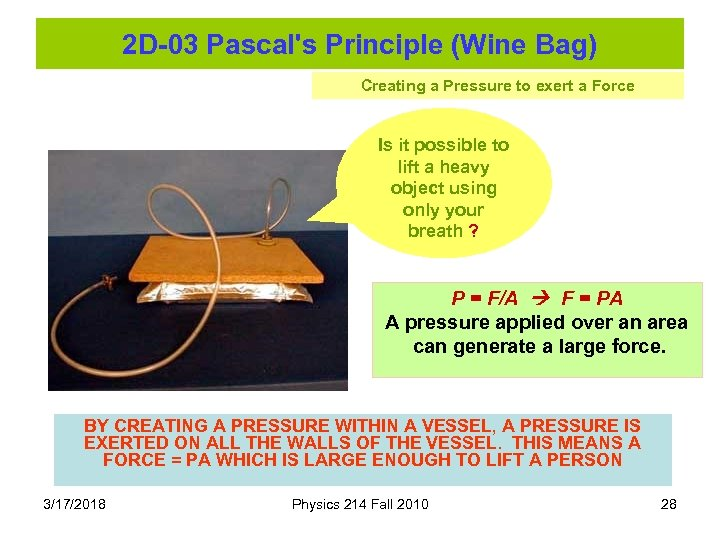2 D-03 Pascal's Principle (Wine Bag) Creating a Pressure to exert a Force Is it possible to lift a heavy object using only your breath ? P = F/A F = PA A pressure applied over an area can generate a large force. BY CREATING A PRESSURE WITHIN A VESSEL, A PRESSURE IS EXERTED ON ALL THE WALLS OF THE VESSEL. THIS MEANS A FORCE = PA WHICH IS LARGE ENOUGH TO LIFT A PERSON 3/17/2018 Physics 214 Fall 2010 28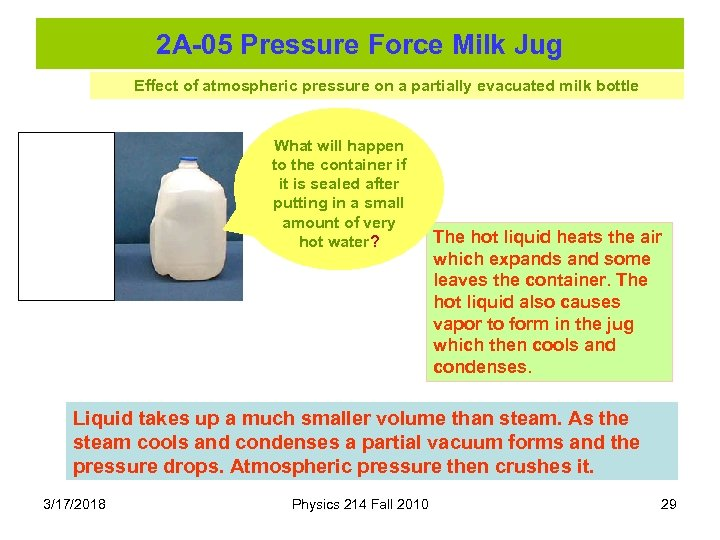2 A-05 Pressure Force Milk Jug Effect of atmospheric pressure on a partially evacuated milk bottle What will happen to the container if it is sealed after putting in a small amount of very hot water? The hot liquid heats the air which expands and some leaves the container. The hot liquid also causes vapor to form in the jug which then cools and condenses. Liquid takes up a much smaller volume than steam. As the steam cools and condenses a partial vacuum forms and the pressure drops. Atmospheric pressure then crushes it. 3/17/2018 Physics 214 Fall 2010 29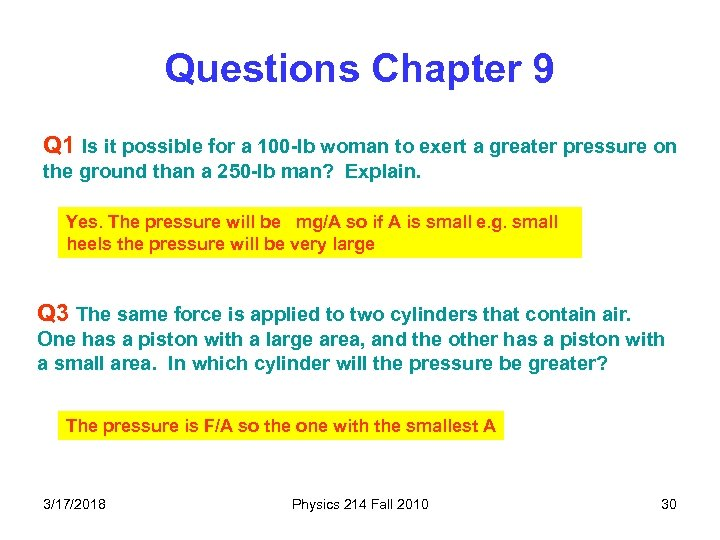Questions Chapter 9 Q 1 Is it possible for a 100 -lb woman to exert a greater pressure on the ground than a 250 -lb man? Explain. Yes. The pressure will be mg/A so if A is small e. g. small heels the pressure will be very large Q 3 The same force is applied to two cylinders that contain air. One has a piston with a large area, and the other has a piston with a small area. In which cylinder will the pressure be greater? The pressure is F/A so the one with the smallest A 3/17/2018 Physics 214 Fall 2010 30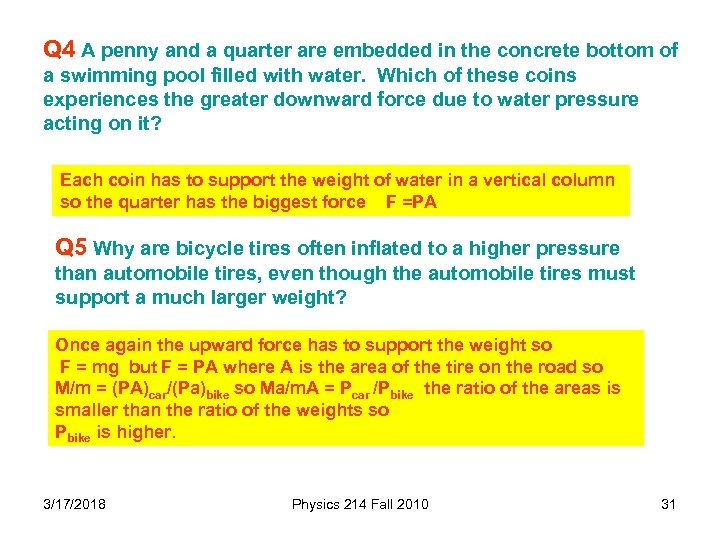Q 4 A penny and a quarter are embedded in the concrete bottom of a swimming pool filled with water. Which of these coins experiences the greater downward force due to water pressure acting on it? Each coin has to support the weight of water in a vertical column so the quarter has the biggest force F =PA Q 5 Why are bicycle tires often inflated to a higher pressure than automobile tires, even though the automobile tires must support a much larger weight? Once again the upward force has to support the weight so F = mg but F = PA where A is the area of the tire on the road so M/m = (PA)car/(Pa)bike so Ma/m. A = Pcar /Pbike the ratio of the areas is smaller than the ratio of the weights so Pbike is higher. 3/17/2018 Physics 214 Fall 2010 31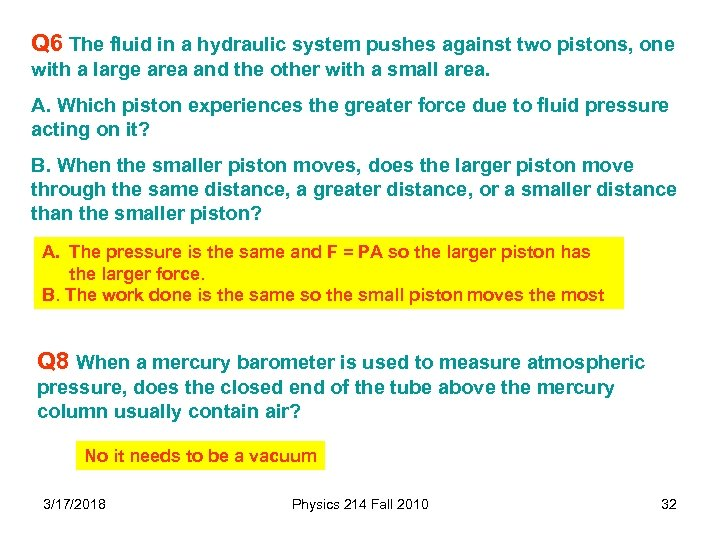Q 6 The fluid in a hydraulic system pushes against two pistons, one with a large area and the other with a small area. A. Which piston experiences the greater force due to fluid pressure acting on it? B. When the smaller piston moves, does the larger piston move through the same distance, a greater distance, or a smaller distance than the smaller piston? A. The pressure is the same and F = PA so the larger piston has the larger force. B. The work done is the same so the small piston moves the most Q 8 When a mercury barometer is used to measure atmospheric pressure, does the closed end of the tube above the mercury column usually contain air? No it needs to be a vacuum 3/17/2018 Physics 214 Fall 2010 32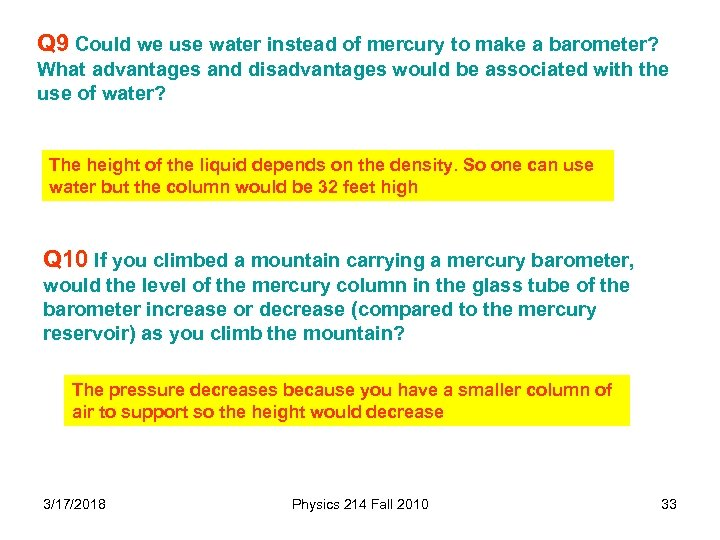Q 9 Could we use water instead of mercury to make a barometer? What advantages and disadvantages would be associated with the use of water? The height of the liquid depends on the density. So one can use water but the column would be 32 feet high Q 10 If you climbed a mountain carrying a mercury barometer, would the level of the mercury column in the glass tube of the barometer increase or decrease (compared to the mercury reservoir) as you climb the mountain? The pressure decreases because you have a smaller column of air to support so the height would decrease 3/17/2018 Physics 214 Fall 2010 33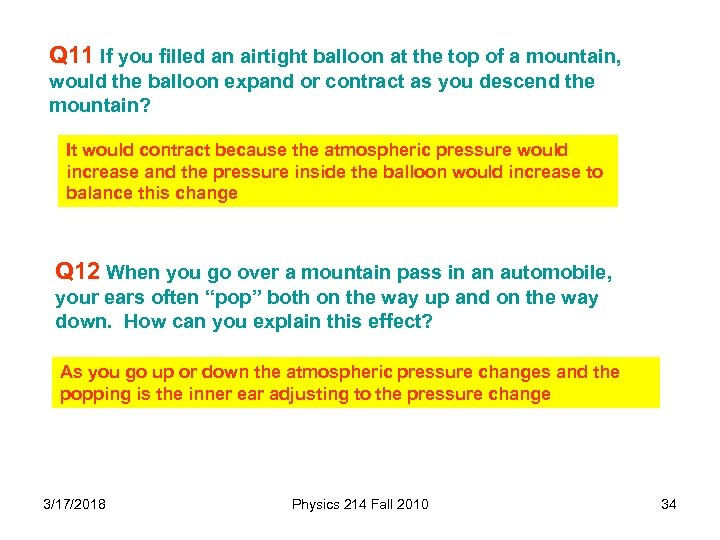Q 11 If you filled an airtight balloon at the top of a mountain, would the balloon expand or contract as you descend the mountain? It would contract because the atmospheric pressure would increase and the pressure inside the balloon would increase to balance this change Q 12 When you go over a mountain pass in an automobile, your ears often “pop” both on the way up and on the way down. How can you explain this effect? As you go up or down the atmospheric pressure changes and the popping is the inner ear adjusting to the pressure change 3/17/2018 Physics 214 Fall 2010 34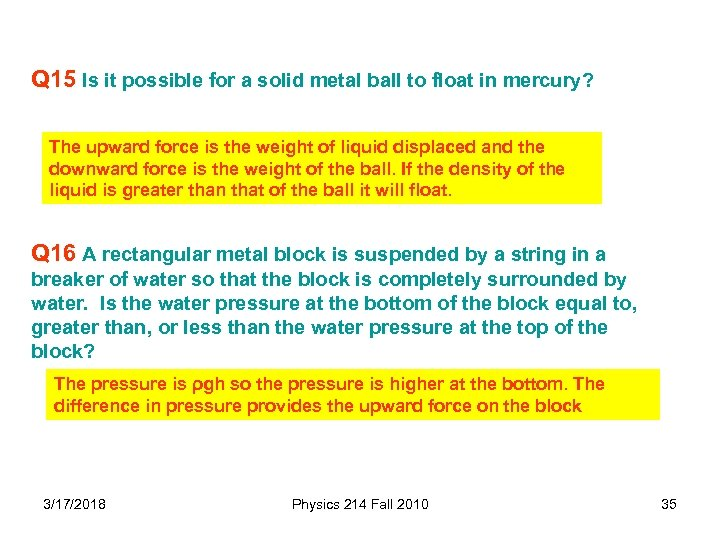Q 15 Is it possible for a solid metal ball to float in mercury? The upward force is the weight of liquid displaced and the downward force is the weight of the ball. If the density of the liquid is greater than that of the ball it will float. Q 16 A rectangular metal block is suspended by a string in a breaker of water so that the block is completely surrounded by water. Is the water pressure at the bottom of the block equal to, greater than, or less than the water pressure at the top of the block? The pressure is ρgh so the pressure is higher at the bottom. The difference in pressure provides the upward force on the block 3/17/2018 Physics 214 Fall 2010 35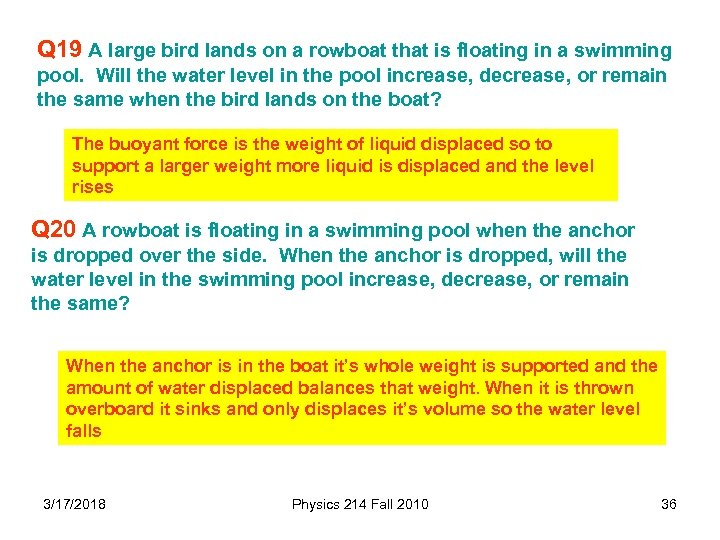Q 19 A large bird lands on a rowboat that is floating in a swimming pool. Will the water level in the pool increase, decrease, or remain the same when the bird lands on the boat? The buoyant force is the weight of liquid displaced so to support a larger weight more liquid is displaced and the level rises Q 20 A rowboat is floating in a swimming pool when the anchor is dropped over the side. When the anchor is dropped, will the water level in the swimming pool increase, decrease, or remain the same? When the anchor is in the boat it’s whole weight is supported and the amount of water displaced balances that weight. When it is thrown overboard it sinks and only displaces it’s volume so the water level falls 3/17/2018 Physics 214 Fall 2010 36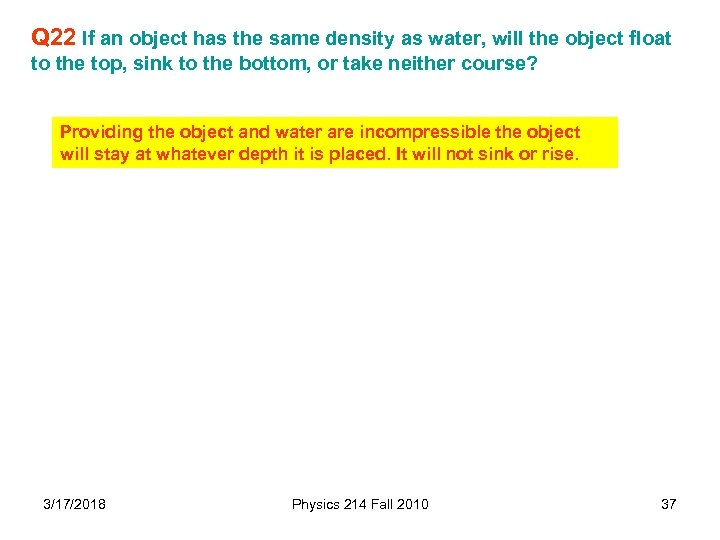Q 22 If an object has the same density as water, will the object float to the top, sink to the bottom, or take neither course? Providing the object and water are incompressible the object will stay at whatever depth it is placed. It will not sink or rise. 3/17/2018 Physics 214 Fall 2010 37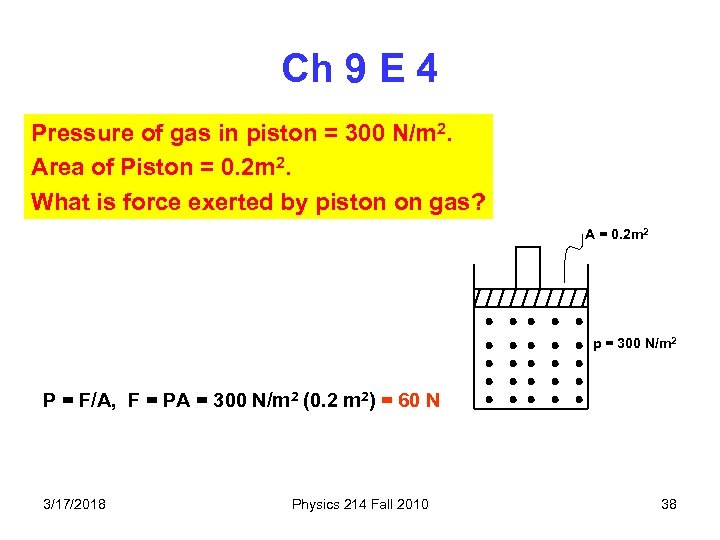Ch 9 E 4 Pressure of gas in piston = 300 N/m 2. Area of Piston = 0. 2 m 2. What is force exerted by piston on gas? A = 0. 2 m 2 p = 300 N/m 2 P = F/A, F = PA = 300 N/m 2 (0. 2 m 2) = 60 N 3/17/2018 Physics 214 Fall 2010 38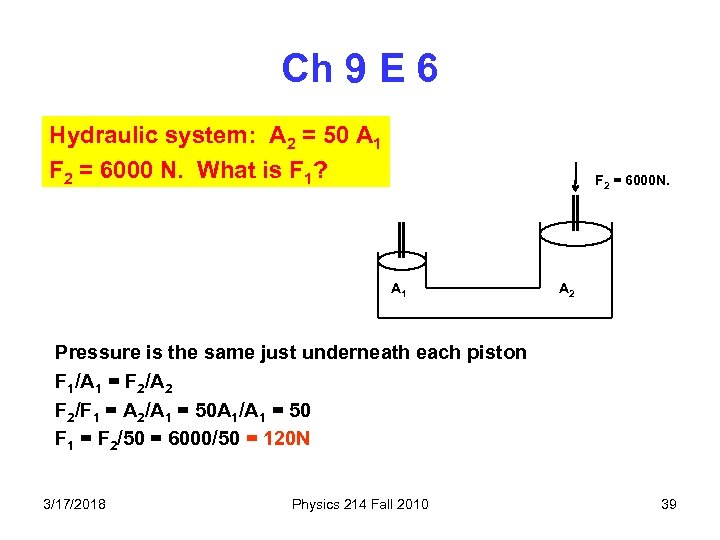Ch 9 E 6 Hydraulic system: A 2 = 50 A 1 F 2 = 6000 N. What is F 1? F 2 = 6000 N. A 1 A 2 Pressure is the same just underneath each piston F 1/A 1 = F 2/A 2 F 2/F 1 = A 2/A 1 = 50 A 1/A 1 = 50 F 1 = F 2/50 = 6000/50 = 120 N 3/17/2018 Physics 214 Fall 2010 39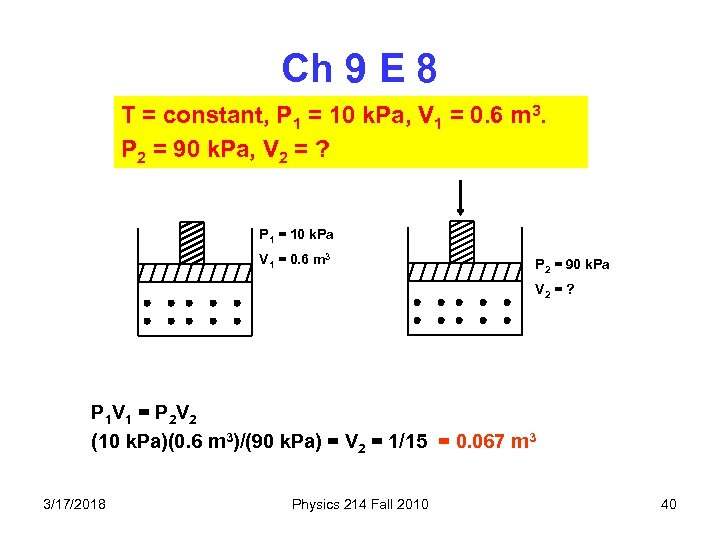Ch 9 E 8 T = constant, P 1 = 10 k. Pa, V 1 = 0. 6 m 3. P 2 = 90 k. Pa, V 2 = ? P 1 = 10 k. Pa V 1 = 0. 6 m 3 P 2 = 90 k. Pa V 2 = ? P 1 V 1 = P 2 V 2 (10 k. Pa)(0. 6 m 3)/(90 k. Pa) = V 2 = 1/15 = 0. 067 m 3 3/17/2018 Physics 214 Fall 2010 40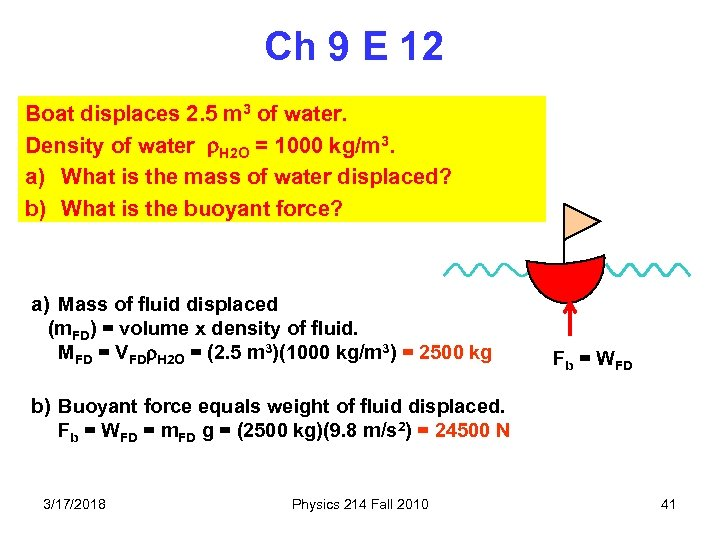Ch 9 E 12 Boat displaces 2. 5 m 3 of water. Density of water H 2 O = 1000 kg/m 3. a) What is the mass of water displaced? b) What is the buoyant force? a) Mass of fluid displaced (m. FD) = volume x density of fluid. MFD = VFD H 2 O = (2. 5 m 3)(1000 kg/m 3) = 2500 kg Fb = WFD b) Buoyant force equals weight of fluid displaced. Fb = WFD = m. FD g = (2500 kg)(9. 8 m/s 2) = 24500 N 3/17/2018 Physics 214 Fall 2010 41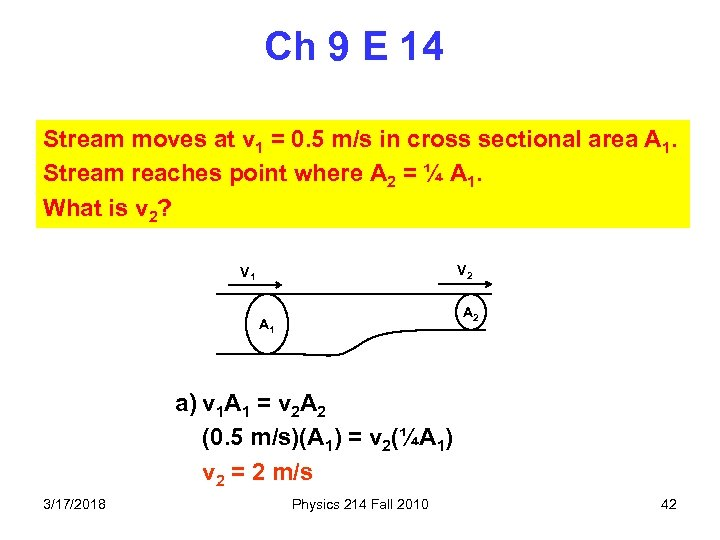Ch 9 E 14 Stream moves at v 1 = 0. 5 m/s in cross sectional area A 1. Stream reaches point where A 2 = ¼ A 1. What is v 2? V 2 V 1 A 2 A 1 a) v 1 A 1 = v 2 A 2 (0. 5 m/s)(A 1) = v 2(¼A 1) v 2 = 2 m/s 3/17/2018 Physics 214 Fall 2010 42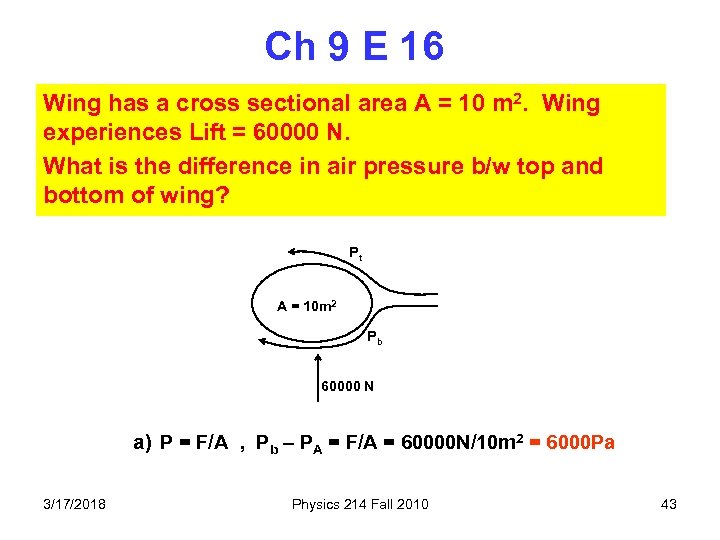Ch 9 E 16 Wing has a cross sectional area A = 10 m 2. Wing experiences Lift = 60000 N. What is the difference in air pressure b/w top and bottom of wing? Pt A = 10 m 2 Pb 60000 N a) P = F/A , Pb – PA = F/A = 60000 N/10 m 2 = 6000 Pa 3/17/2018 Physics 214 Fall 2010 43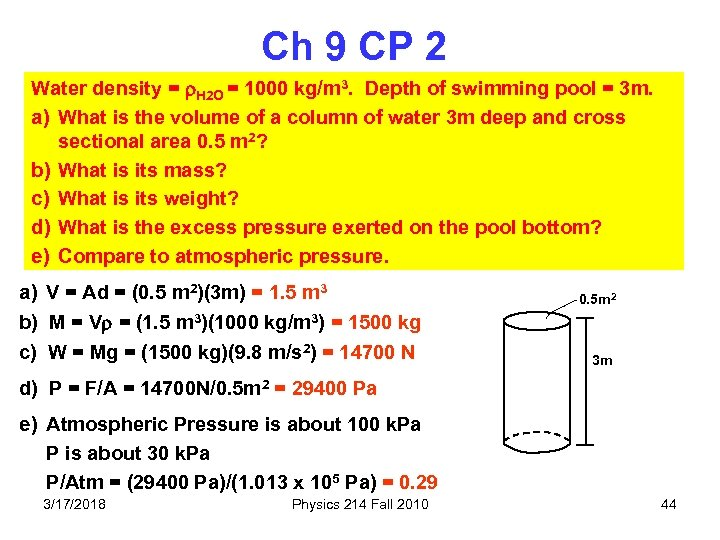Ch 9 CP 2 Water density = H 2 O = 1000 kg/m 3. Depth of swimming pool = 3 m. a) What is the volume of a column of water 3 m deep and cross sectional area 0. 5 m 2? b) What is its mass? c) What is its weight? d) What is the excess pressure exerted on the pool bottom? e) Compare to atmospheric pressure. a) V = Ad = (0. 5 m 2)(3 m) = 1. 5 m 3 b) M = V = (1. 5 m 3)(1000 kg/m 3) = 1500 kg c) W = Mg = (1500 kg)(9. 8 m/s 2) = 14700 N 0. 5 m 2 3 m d) P = F/A = 14700 N/0. 5 m 2 = 29400 Pa e) Atmospheric Pressure is about 100 k. Pa P is about 30 k. Pa P/Atm = (29400 Pa)/(1. 013 x 105 Pa) = 0. 29 3/17/2018 Physics 214 Fall 2010 44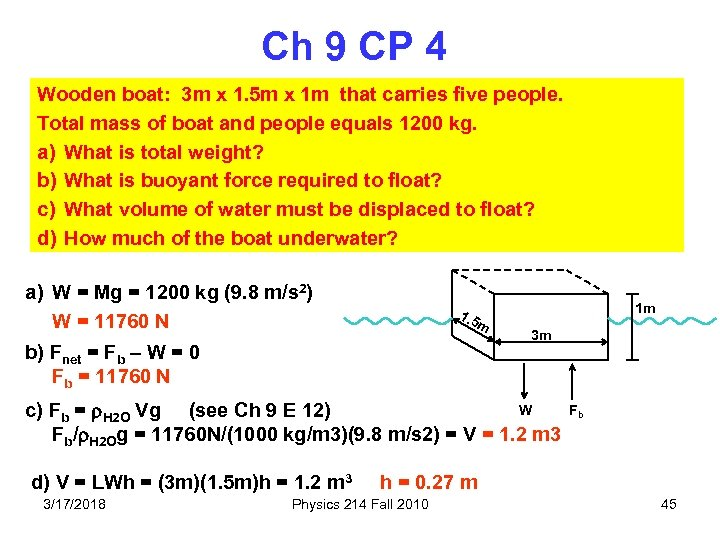Ch 9 CP 4 Wooden boat: 3 m x 1. 5 m x 1 m that carries five people. Total mass of boat and people equals 1200 kg. a) What is total weight? b) What is buoyant force required to float? c) What volume of water must be displaced to float? d) How much of the boat underwater? a) W = Mg = 1200 kg (9. 8 m/s 2) W = 11760 N 1. 5 m b) Fnet = Fb – W = 0 Fb = 11760 N 1 m 3 m W c) Fb = H 2 O Vg (see Ch 9 E 12) Fb/ H 2 Og = 11760 N/(1000 kg/m 3)(9. 8 m/s 2) = V = 1. 2 m 3 d) V = LWh = (3 m)(1. 5 m)h = 1. 2 m 3 3/17/2018 Fb h = 0. 27 m Physics 214 Fall 2010 45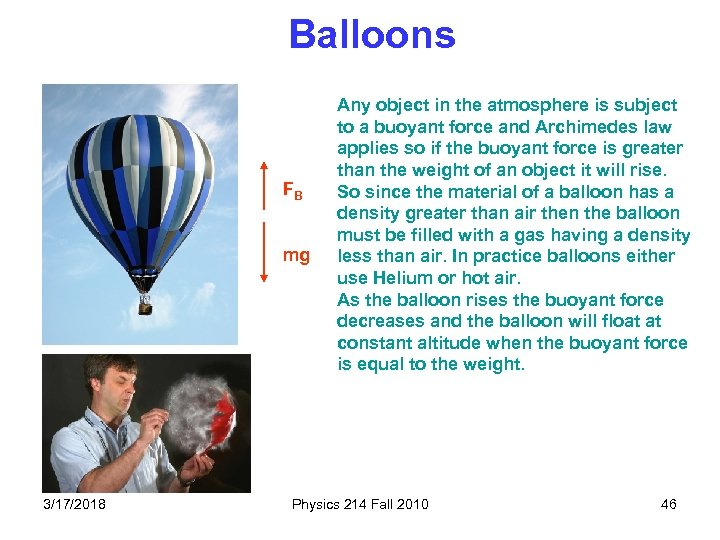Balloons FB mg 3/17/2018 Any object in the atmosphere is subject to a buoyant force and Archimedes law applies so if the buoyant force is greater than the weight of an object it will rise. So since the material of a balloon has a density greater than air then the balloon must be filled with a gas having a density less than air. In practice balloons either use Helium or hot air. As the balloon rises the buoyant force decreases and the balloon will float at constant altitude when the buoyant force is equal to the weight. Physics 214 Fall 2010 46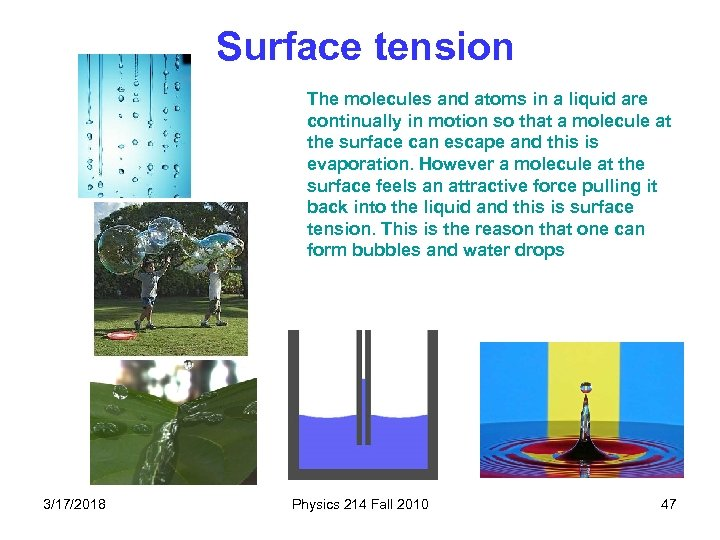Surface tension The molecules and atoms in a liquid are continually in motion so that a molecule at the surface can escape and this is evaporation. However a molecule at the surface feels an attractive force pulling it back into the liquid and this is surface tension. This is the reason that one can form bubbles and water drops 3/17/2018 Physics 214 Fall 2010 47